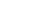xAmpere-turnEncyclopedia
The ampere-turn was the MKS
Mks system of units
The MKS system of units is a physical system of units that expresses any given measurement using fundamental units of the metre, kilogram, and/or second ....

unit of magnetomotive force
Magnetomotive force
Magnetomotive force is any physical driving force that produces magnetic flux. In this context, the expression "driving force" is used in a general sense of "work potential", and is analogous, but distinct from force measured in newtons...

(MMF), represented by a direct current
Direct current
Direct current is the unidirectional flow of electric charge. Direct current is produced by such sources as batteries, thermocouples, solar cells, and commutator-type electric machines of the dynamo type. Direct current may flow in a conductor such as a wire, but can also flow through...

of one ampere
Ampere
The ampere , often shortened to amp, is the SI unit of electric current and is one of the seven SI base units. It is named after André-Marie Ampère , French mathematician and physicist, considered the father of electrodynamics...

flowing in a single-turn loop in a vacuum. "Turns
Turn (geometry)
A turn is an angle equal to a 360° or 2 radians or \tau radians. A turn is also referred to as a revolution or complete rotation or full circle or cycle or rev or rot....

" refers to the winding number
Winding number
In mathematics, the winding number of a closed curve in the plane around a given point is an integer representing the total number of times that curve travels counterclockwise around the point...

of an electrical conductor comprising an inductor.

The ampere-turn was replaced by the SI unit, ampere
Ampere
The ampere , often shortened to amp, is the SI unit of electric current and is one of the seven SI base units. It is named after André-Marie Ampère , French mathematician and physicist, considered the father of electrodynamics...

.

For example, a current of 2A flowing through a coil of 10 turns produces an MMF of 20 AT.

Here we see that by maintaining the same current and simply increasing the number of loops or turns of the coil, the strength of the magnetic field increases. This is because each loop or turn of the coil sets up its own magnetic field, which unites with the fields of the other loops to produce the field around the entire coil. The more loops, the more magnetic fields unite and reinforce each other and, as a result, the total magnetic field becomes stronger.

The strength of the magnetic field is not linearly related to the ampere turns when a magnetic material is used as a part of the system. Also, the material within the magnet carrying the magnetic flux "saturates" at some point, when adding more ampere turns has little effect.

The ampere-turn is equal togilbert
Magnetomotive force
Magnetomotive force is any physical driving force that produces magnetic flux. In this context, the expression "driving force" is used in a general sense of "work potential", and is analogous, but distinct from force measured in newtons...

s, the equivalent CGS unit.